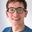# Mambu Banking Engine

## Act/Act Day Count Convention for Deposit Accounts

What

The introduction of the Act/Act day count convention. This would mean that the actual number of days in the period divided by the number of days in the current year are used. For example:

01.01.19 to 01.02.19 = 31/365

01.02.19 to 01.03.19 = 28/365

01.01.20 to 01.02.20 = 31/366

01.02.20 to 01.03.20 = 29/366

This is also mentioned briefly here: http://www.deltaquants.com/day-count-conventions

Why

Some organisations require interest calculations which take the real number of days in both the given period and year into account.

•James Anderson
• Nov 30 2018
• Shipped
•